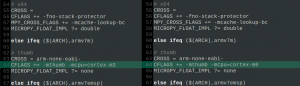# 在Raspberry Pi Pico上为MicroPython提供C语言扩展

## 简介

### MicroPython C语言扩展的类型

.py是一个存储已编译的本地机器代码的文件，可以动态链接。这使得它更加灵活，因为你不需要重新编译整个MicroPython固件。

## 编写扩展模块

cd ~/Desktop/factfib

``````#include "py/dynruntime.h

STATIC mp_int_t factorial_helper(mp_int_t x) {
如果(x < 1) {
返回1。
}
return x*factorial_helper(x-1);
}

STATIC mp_obj_t factorial(mp_obj_t x_obj) {
mp_int_t x = mp_obj_get_int(x_obj);
mp_int_t rslt = factorial_helper(x);
return rslt;
}

STATIC MP_DEFINE_CONST_FUN_OBJ_1(factorial_obj, factorial)。

STATIC mp_int_t fibonacci_helper(mp_int_t x) {
如果(x <= 0) {
返回0。
}
否则如果(x == 1) {
返回1。
}
否则 {
返回（fibonacci_helper(x-1) + fibonacci_helper(x-2)）。
}
}

STATIC mp_obj_t fibonacci(mp_obj_t x_obj) {
mp_int_t x = mp_obj_get_int(x_obj);
mp_int_t rslt = fibonacci_helper(x);
return mp_obj_new_int(rslt);
}

STATIC MP_DEFINE_CONST_FUN_OBJ_1(fibonacci_obj, fibonacci) 。

mp_obj_t mpy_init(mp_obj_fun_bc_t *self, size_t n_args, size_t n_kw, mp_obj_t *args) {
mp_dynruntime_init_entry

mp_store_global(MP_QSTR_factorial, MP_OBJ_FROM_PTR(&factorial_obj))。
mp_store_global(MP_QSTR_fibonacci, MP_OBJ_FROM_PTR(&fibonacci_obj))。

mp_dynruntime_init_exit
}``````

## 构建扩展

sudo apt-get install build-essential libreadline-dev libffi-dev git pkg-config gcc-arm-one-eabi libnewlib-arm-one-eabi

git clone -recurse-submodules https://github.com/micropython/micropython.git

pip3 安装 'pyelftools>=0.25'

``````MPY_DIR = micropython

MOD = factfib

SRC = factfib.c

ARCH = armv7m

include \$(MPY_DIR)/py/dynruntime.mk``````## 安装并测试该扩展

sudo pip3 install rshell

rshell -version

rshell -p /dev/ttyACM0 -buffer-size 512

cp factfib.mpy /pyboard/factfib.mpy

sudo apt install minicom

minicom -o -D /dev/ttyACM0

>>>导入 factfib 作为 ff

>>> ff.阶乘(5)

>>> 120

>> ff.fibonacci(7)

>>> 13

### 2评论

1. PeterB 在11 月 26, 2021在8:29 上午

除了c程序中的错误外，它工作得很好。谢谢。

STATIC mp_obj_t factorial(mp_obj_t x_obj) {
mp_int_t x = mp_obj_get_int(x_obj)。
mp_int_t rslt = factorial_helper(x);
返回mp_obj_new_int(rslt)。
}